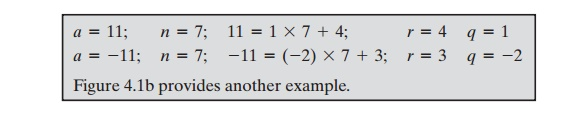Home | | Cryptography and Network Security | Divisibility and the Division Algorithm

# Divisibility and the Division Algorithm

We say that a nonzero b divides a if a = mb for some m, where a, b, and m are integers. That is, b divides a if there is no remainder on division.

DIVISIBILITY AND THE DIVISION ALGORITHM

Divisibility

We say that a nonzero b divides a if a = mb for some m, where a, b, and m are  integers. That is, b divides a if there is no remainder on division. The notation b | a is commonly used to mean b divides a. Also, if b | a, we say that b is a divisor of a.Subsequently, we will need some simple properties of divisibility for integers, which are as follows:The Division Algorithm

Given any positive integer n and any nonnegative integer a, if we divide a by n, we get an integer quotient q and an integer remainder r that obey the following relationship:where |x | is the largest integer less than or equal to x. Equation (4.1) is referred to as the division algorithm.

Figure 4.1a demonstrates that, given a and positive n, it is always possible to find q and r that satisfy the preceding relationship. Represent the integers on the number line; a will fall somewhere on that line (positive a is shown, a similar demonstration can be made for negative a). Starting at 0, proceed to n, 2n, up to qn, such that qn <=a and(q + 1)n > a. The distance from qn to a is r, and we have found the unique values of q and r. The remainder r is often referred to as a residue.Study Material, Lecturing Notes, Assignment, Reference, Wiki description explanation, brief detail
Cryptography and Network Security Principles and Practice : One Symmetric Ciphers : Basic Concepts in Number Theory and Finite Fields : Divisibility and the Division Algorithm |